# How to merge two csv files by specific column using Pandas in Python?

• Difficulty Level : Basic
• Last Updated : 13 Jan, 2021

In this article, we are going to discuss how to merge two CSV files there is a function in pandas library pandas.merge(). Merging means nothing but combining two datasets together into one based on common attributes or column.

Syntax: pandas.merge()

Attention geek! Strengthen your foundations with the Python Programming Foundation Course and learn the basics.

To begin with, your interview preparations Enhance your Data Structures concepts with the Python DS Course. And to begin with your Machine Learning Journey, join the Machine Learning - Basic Level Course

Parameters :

• data1, data2: Dataframes used for merging.
• how: {‘left’, ‘right’, ‘outer’, ‘inner’}, default ‘inner’
• on: label or list

Returns : A DataFrame of the two merged objects.

### There are 4 types of a merge.

• Inner
• Left
• Right
• Outer

We are going to use the below two csv files i.e. loan.csv and borrower.csv to perform all operations: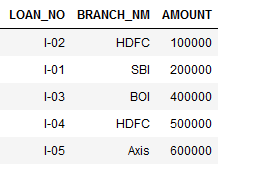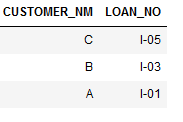### Inner Join

By setting how=’inner‘ it will merge both dataframes based on the specified column and then return new dataframe containing only those rows that have a matching value in both original dataframes.Code:

## Python3

 `import` `pandas as pd`` ` `# reading two csv files``data1 ``=` `pd.read_csv(``'datasets/loan.csv'``)``data2 ``=` `pd.read_csv(``'datasets/borrower.csv'``)`` ` `# using merge function by setting how='inner'``output1 ``=` `pd.merge(data1, data2, ``                   ``on``=``'LOAN_NO'``, ``                   ``how``=``'inner'``)`` ` `# displaying result``print``(output1)`

Output: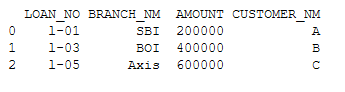### Left Outer Join

By setting how=’left’ it will merge both dataframes based on the specified column and then return new dataframe containing all rows from left dataframe including those rows also who do not have values in the right dataframe and set right dataframe column value to NAN.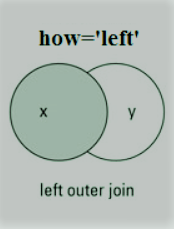Code:

## Python3

 `import` `pandas as pd`` ` `# reading csv files``data1 ``=` `pd.read_csv(``'datasets/loan.csv'``)``data2 ``=` `pd.read_csv(``'datasets/borrower.csv'``)`` ` `# using merge function by setting how='left'``output2 ``=` `pd.merge(data1, data2, ``                   ``on``=``'LOAN_NO'``, ``                   ``how``=``'left'``)`` ` `# displaying result``print``(output2)`

Output: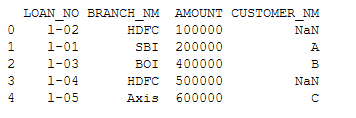### Right Outer Join

By setting how=’right’ it will merge both dataframes based on the specified column and then return new dataframe containing all rows from right dataframe including those rows also who do not have values in the left dataframe and set left dataframe column value to NAN.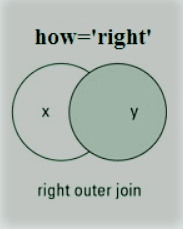Code:

## Python3

 `import` `pandas as pd`` ` `# reading csv files``data1 ``=` `pd.read_csv(``'datasets/loan.csv'``)``data2 ``=` `pd.read_csv(``'datasets/borrower.csv'``)`` ` `# using merge function by setting how='right'``output3 ``=` `pd.merge(data1, data2,``                   ``on``=``'LOAN_NO'``,``                   ``how``=``'right'``)`` ` `# displaying result``print``(output3)`

Output:### Full Outer Join

By setting how=’right’ it will merge both dataframes based on the specified column and then return new dataframe containing rows from both dataframes and set NAN value for those where data is missing in one of the dataframes.Code:

## Python3

 `import` `pandas as pd`` ` `# reading csv files``data1 ``=` `pd.read_csv(``'datasets/loan.csv'``)``data2 ``=` `pd.read_csv(``'datasets/borrower.csv'``)`` ` `# using merge function by setting how='outer'``output4 ``=` `pd.merge(data1, data2, ``                   ``on``=``'LOAN_NO'``, ``                   ``how``=``'outer'``)`` ` `# displaying result``print``(output4)`

Output: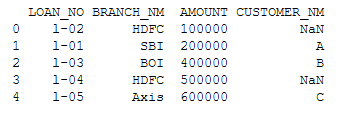My Personal Notes arrow_drop_up